# The study of functions, graphing functions

Example.1. Scope
2.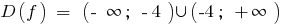3. Parity, odd parity, periodicity
4. The function is neither even nor odd and periodic

5. The intersection with the coordinate axes
6.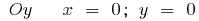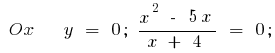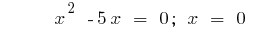or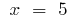7. The derivative and critical points
8.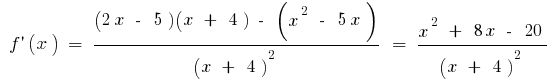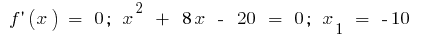or9. Intervals of increase, decrease, and extreme points (and the value of the function at these points)
10.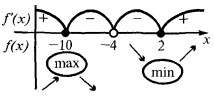11. The behavior of the functions at the ends of the scope and the asymptotes of the graph of a function (vertical, horizontal and inclined)When you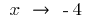left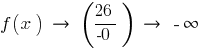Inthe case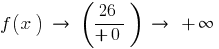Therefore,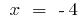vertical asymptote

Since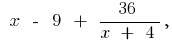whenthen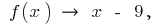ie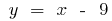is the oblique asymptote

12. The second derivative and the study of the function of the bulge and gnutt
13. Find the inflection points (if they exist) and valuesin the points of inflection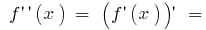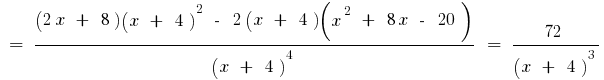So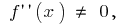the sign of the second derivative can change only at the point14. If you want to find control points specifying the behavior of the graph
15.16. On the basis of the study building sketch the graph of a function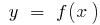17.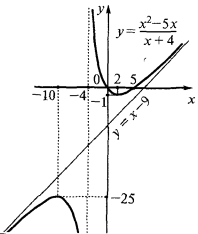Tags:
Chapter:
Versions in other languages:
Share with friends: